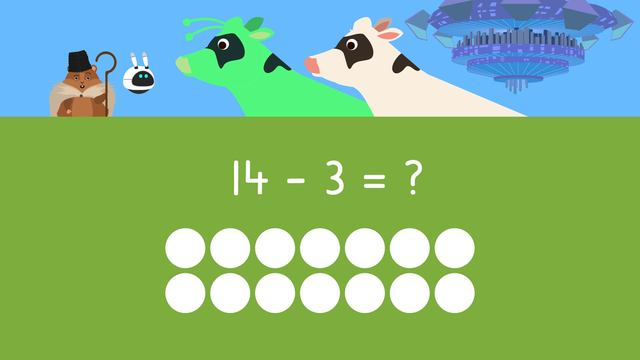# Subtraction Word ProblemsRating

Ø 5.0 / 1 ratings

The authorsTeam Digital
Subtraction Word Problems
CCSS.MATH.CONTENT.1.OA.A.1

## Basics on the topicSubtraction Word Problems

### Content

In this subtraction word problems 1st grade video, Mr. Squeaks and Imani are looking after some cows in the meadow. When some decide to leave to the watering hole to get a drink, we need to figure out how many are left by solving math subtraction word problems one step subtraction word problems. Let’s help them by learning how to solve one step subtraction word problems.

### What is Subtraction Word Problems

Subtraction word problems grade 1 are simple subtraction word problems that ask the learner to solve by first reading or listening to the word problem, understanding the information from the subtraction word problems for 1st grade, and then asks them to solve for the answer to word problems with subtraction.

What is an example of a subtraction word problem? In the next section we will look at an example of word problems subtraction.

#### First Grade Subtraction Word Problems Example

This section will explain how to solve subtraction word problems by looking at basic subtraction word problems. Let’s begin:

The first step is to read or listen to the word problem. As you read or listen, think; what do I need to figure out?’, and highlight the question you need to solve by looking for the question mark.Here we highlight “How many are left?”,because that's what we need to figure out!The second step is to read or listen to the word problem again and highlight the clue words and numbers. Clue words are words that tell us the important information we need to figure out the answer! Here, we highlight, "fourteen cows”, because that's how many cows were there at first. We also highlight, "three cows leave", because that tells us how many left to drink water.The third step is to think: Do I add or subtract? How do you know when to subtract in a word problem? There are certain words that mean subtraction in word problems! In this subtraction word problem, "Three cows leave", tells us to subtract because they are going away!

The fourth step is to write a number sentence. The number sentence we need to solve is fourteen minus three equals question mark, because there were fourteen cows, and three left to go to the watering hole, so we figure out fourteen minus three to see how many are left!The last step is to solve the number sentence. We can draw circles to help us solve, so we have fourteen circles. Since we are subtracting three, we will cross off three. How many are left?Fourteen minus three equals eleven!

### Subtraction Word Problems Summary

How do you solve word problems with subtraction? Remember, to find the answer to a word problem using subtraction:

• First, read or listen to the word problem. As you read, think; ‘what do I need to figure out?’, and highlight the question you need to solve by looking for the question mark
• Next, read or listen to it again and highlight the clue words and numbers
• Then, think: ‘Do I add or subtract?’